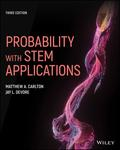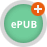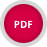John Wiley & Sons Probability with STEM ApplicationsProbability with STEM Applications, Third Edition, is an accessible and well-balanced introduction t.. Product #: 978-1-119-71786-7 Regular price: \$111.21 \$111.21 Auf Lager

# Probability with STEM Applications3. Auflage März 2021
640 Seiten, Softcover
Wiley & Sons Ltd

ISBN: 978-1-119-71786-7
John Wiley & Sons

Jetzt kaufen

### Preis: 119,00 €

Preis inkl. MwSt, zzgl. Versand

Weitere VersionenProbability with STEM Applications, Third Edition, is an accessible and well-balanced introduction to post-calculus applied probability. Integrating foundational mathematical theory and the application of probability in the real world, this leading textbook engages students with unique problem scenarios and more than 1100 exercises of varying levels of difficulty. The text uses a hands-on, software-oriented approach to the subject of probability. MATLAB and R examples and exercises -- complemented by computer code that enables students to create their own simulations -- demonstrate the importance of software to solve problems that cannot be obtained analytically.

Revised and updated throughout, the textbook covers random variables and probability distributions, the basics of statistical inference, Markov chains, stochastic processes, signal processing, and more. This new edition is the perfect text for both year-long and single-semester mathematics and statistics courses, student engineers and scientists, and business and social science majors wanting to learn the quantitative aspects of their disciplines.

Preface

Introduction

Chapter 1: Introduction to Probability

Chapter 2: Conditional Probability and Independence

Chapter 3: Discrete Probability Distributions: General Properties

Chapter 4: Families of Discrete Distributions

Chapter 5: Continuous Probability Distributions: General Properties

Chapter 6: Families of Continuous Distributions

Chapter 7: Joint Probability Distributions

Chapter 8: Joint Probability Distributions: Additional Topics

Chapter 9: The Basics of Statistical Inference

Chapter 10: Markov Chains

Chapter 11: Random Processes

Chapter 12: Families of Random Processes

Chapter 13: Introduction to Signal Processing

Appendix A: Statistical Tables

Appendix B: Background Mathematics

Appendix C: Important Probability Distributions

Solutions to Odd-Numbered Exercises

References

Index